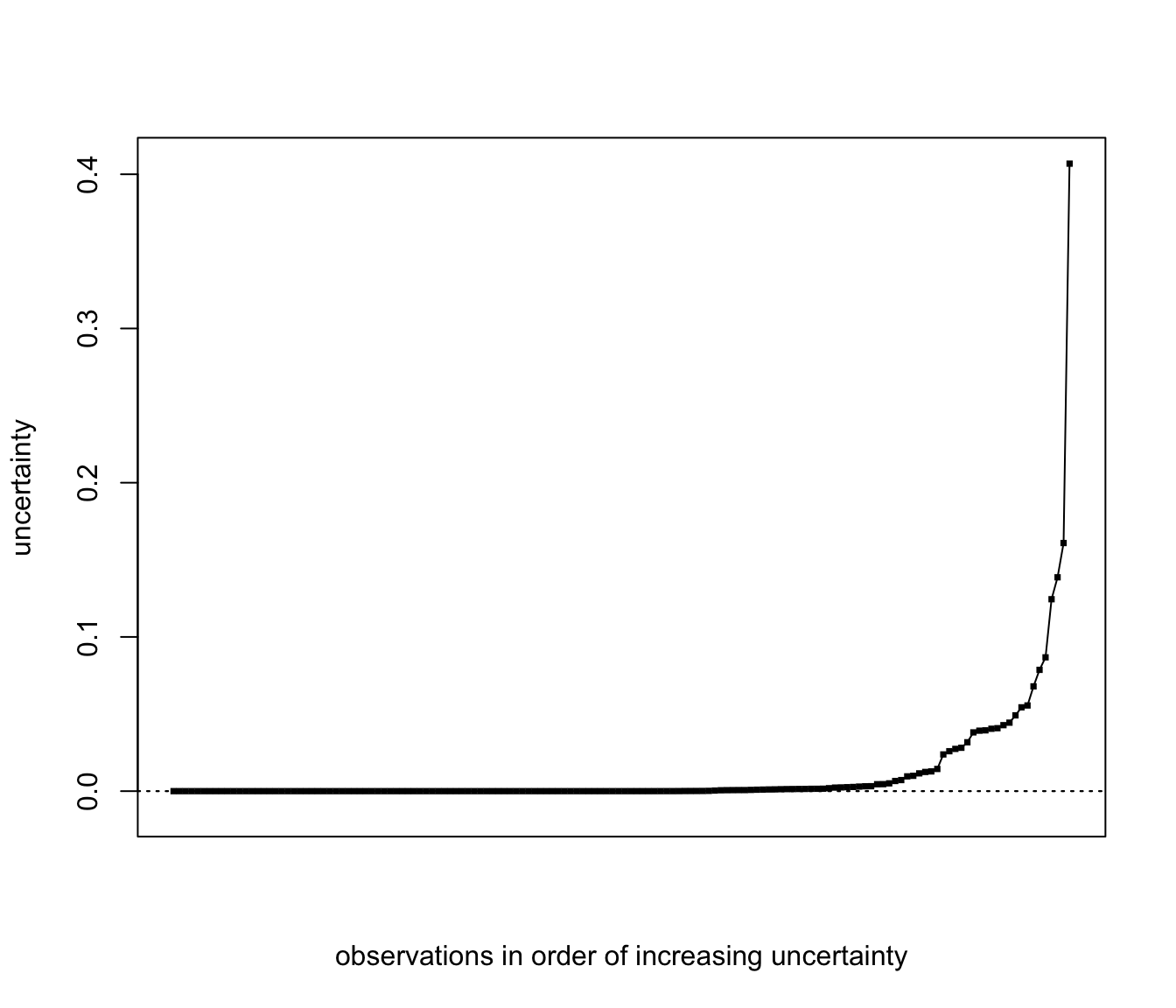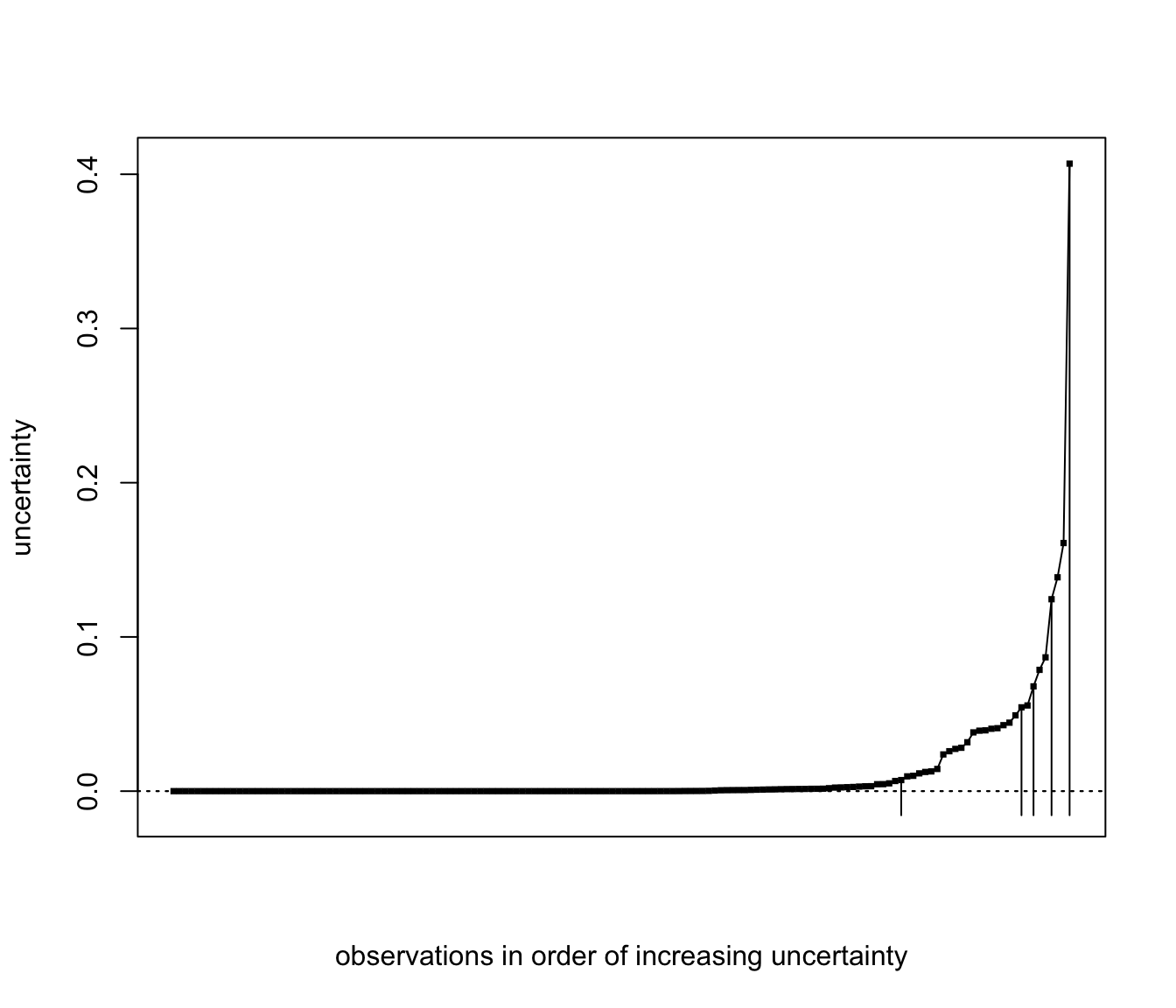Displays the uncertainty in converting a conditional probablility from EM to a classification in model-based clustering.

uncerPlot(z, truth, ...)

Arguments

z

A matrix whose [i,k]th entry is the conditional probability of the ith observation belonging to the kth component of the mixture.

truth

A numeric or character vector giving the true classification of the data.

...

Provided to allow lists with elements other than the arguments can be passed in indirect or list calls with do.call.

Value

A plot of the uncertainty profile of the data, with uncertainties in increasing order of magnitude. If truth is supplied and the number of classes is the same as the number of columns of z, the uncertainty of the misclassified data is marked by vertical lines on the plot.

Details

When truth is provided and the number of classes is compatible with z, the function compareClass is used to to find best correspondence between classes in truth and z.

uncerPlot(z = irisModel3$z)uncerPlot(z = irisModel3$z, truth = iris[,5])